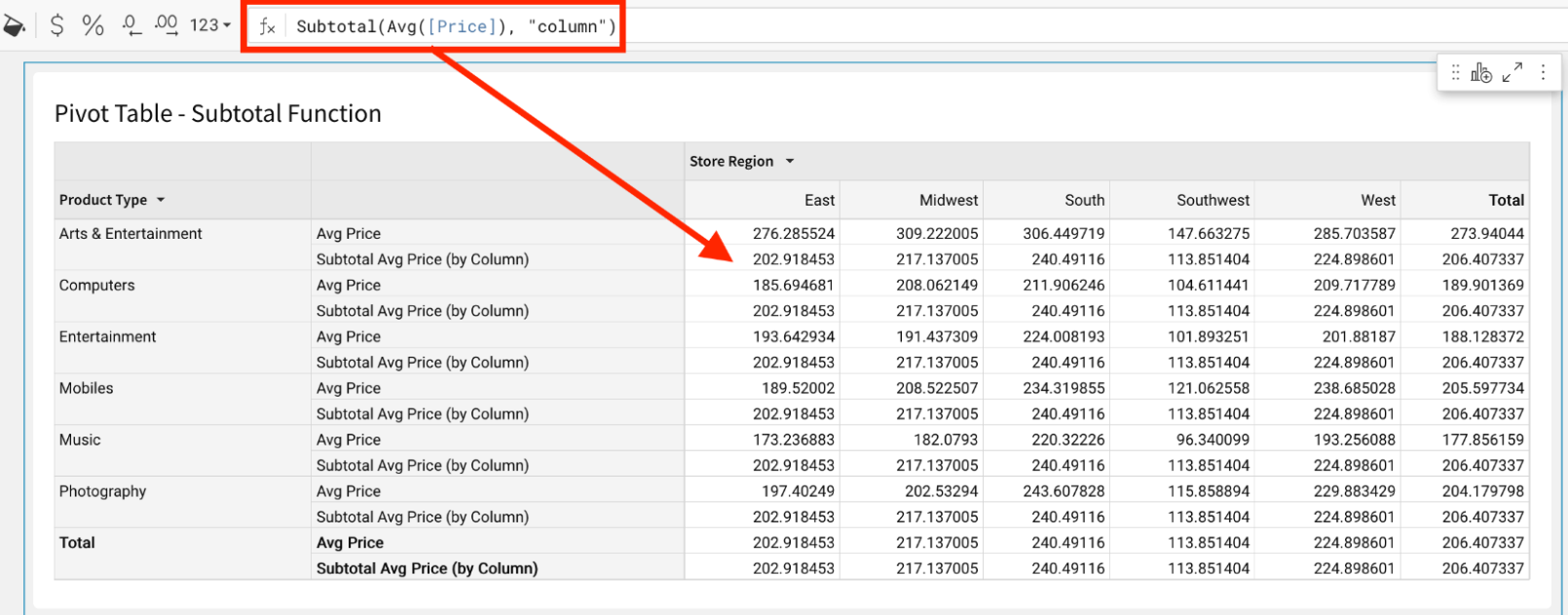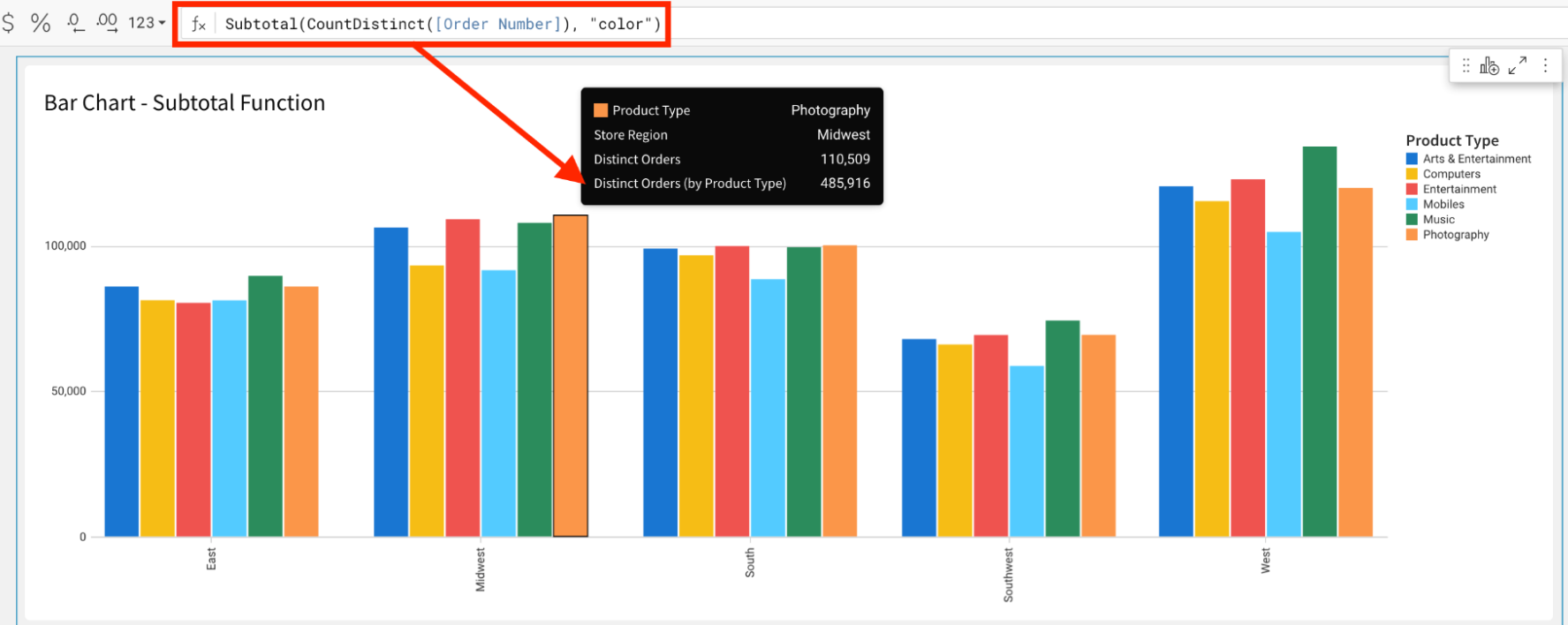# Subtotal

The Subtotal function returns the subtotal for an aggregate formula.

## Syntax

`Subtotal(aggregate, mode, [parameters])`

Function arguments:

• aggregate (required) - The aggregate formula to use
• mode (required) - The mode to use when calculating the aggregate formula. Mode determines which dimension(s) to use when performing the calculation.
• General purpose modes
• `"grand_total"` (default for visualizations and pivot tables) - Calculates the subtotal for the aggregate formula.
• Visualization modes
• `"color"` - Calculates the aggregate formula using only the column specified for COLOR.
• `"x_axis"` - Calculates the aggregate formula using only the column(s) placed on the X-AXIS.
• `"trellis_column"` / `"trellis_row"` - Calculates the aggregate subtotal using only the dimension specified for the TRELLIS COLUMN or TRELLIS ROW.
• Pivot table modes
• `"column"` / `"row"` - Calculates aggregate subtotal for the column or row total.
• `"column_parent"` / `"row_parent"` - Calculates the aggregate subtotal for the column or row total, relative to the subtotal calculation for the parent dimensions.
• Table modes
• `"parent_grouping"` - Calculates the aggregate formula using the grouping keys from a parent grouping. This is equivalent to creating the aggregate calculation in a parent grouping and referencing it from a column in a lower grouping.
• parameters (optional) - An additional parameter specific to the mode.
• This is only applicable to the following modes: "column_parent", "row_parent" and "parent_grouping".  For these modes, this 3rd parameter specifies how many parent dimensions to ignore.

## Examples

`Subtotal(Avg([Price]), "column")`
• Calculates the aggregate subtotal for each column of the Product Type dimension.
• Verify that the calculation values match the built-in pivot subtotals at the bottom of the table.`Subtotal(CountDistinct([Product Type]), "x_axis")`
• Calculates the aggregate subtotal for each Product Type, across all values of Store Region.
• The calculation is shown in the tooltip, but is usable anywhere an aggregate formula is allowed.